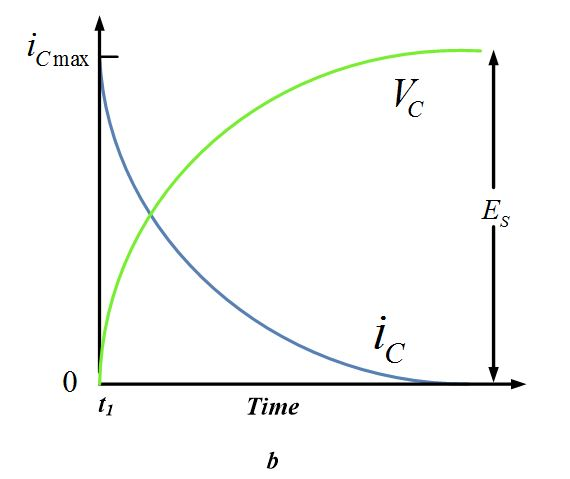# RC Circuit Time Constant | Charging Discharging of Capacitor

Want create site? Find Free WordPress Themes and plugins.

Definition:The time required to charge a capacitor to about 63 percent of the maximum voltage in an RC circuit is called the time constant of the circuit.

When a discharged capacitor is suddenly connected across a DC supply, such as Es in figure 1 (a), a current immediately begins to flow. At time t1 (Figure 1 (b)), the moment the circuit is closed, the capacitor acts like a short circuit. The in-rush current iC is at its maximum value and is limited only by the series resistor R. however, as soon as the capacitor begins o charge, electrons from the supply being to build upon the lower plate of the capacitor. Simultaneously, electrons from the upper plate are attracted to the positive terminal of the supply. A difference of potential now begins to appear across the capacitor. The polarity, being the same as that of the source, opposes Es.

## Exponential Curve

A curve that varies by the square or some other power of a factor instead of linearity.

The net voltage available to charge the capacitor is the difference between the capacitor voltage vC and Es. (Note: lowercase letters are used to designate voltage and current values that are changing). As VC, capacitor’s emf increases, this net voltage diminishes. Consequently, the rate of charge slows down. This cumulative effect continues until VC is approximately equal to Es, at which time the charging current iC is reduced to nearly zero. See figure 1 (b). Theoretically, the capacitor never reaches a full charge, but most capacitors can be considered fully charged in several seconds or less. Curves iC and VC in figure 1 (b) are examples of exponential curves. An exponential curve has the property of dropping or rising very quickly toward a limiting value. The closer it goes to the limit, the more gradual its approach becomes.Fig.1: Effect in an RC Circuit: (a) RC Circuit (b) Current and Voltage Waveforms for Closed Switch

## Effect of R and C on Charging Time

Inasmuch as the charging current must flow through the series resistor, this current inevitably has an effect on the rate of charge.

Changing Resistance

If the resistance is increased (C unchanged), the IR drop produced by the charging current in greater, and the net voltage charging the capacitor is reduced. Therefore, the capacitor takes a longer period of time to reach full charge when the series resistance in increased. Of course, the reverse is true if R is made smaller.

Changing Capacitance

Consider what happens when the series resistance remains constant but the capacitance increases. The initial in-rush of current is the same as illustrated in figure 1, assuming the same supply voltage. Since the capacitor’s charge capacity Q is equal to CV, it is apparent that more time will be required to charge that capacitor because C is larger. The emf across the capacitor builds up more slowly, causing the rate of charge to be slowed down. Hence, the charging time of a capacitor is directly proportional to its capacitance.

## Time constant $\tau =RC$

Whenever a voltage or current constantly changes value, it exhibits transient effects. The voltage across the resistance and capacitance in an RC circuit have these characteristics. They are of a transient nature until reaching steady-state values.

## Time constant τ

In a capacitor, the time required for a voltage to reach 63.2 % of the steady-state or full charge value. In an inductor, the time required for a current to reach 63.2 % of full or steady-state value.

When analyzing the amount of time it takes an RC circuit to reach a steady state condition, we must deal with a term referred to as circuit’s time constant. Expressed mathematically, the time constant τ is as follows:

$\tau =RC$

The time constant τ (Greek lowercase letter tau) is expressed in seconds when R is in ohms and C is in farads. That τ is expressed in seconds can be derived as follows:

$\tau =R*C=ohms*farads=\frac{coulombs}{volts}*\frac{volts}{amperes}$

$\text{ }\tau \text{ =}\frac{\text{coulombs}}{\text{amperes}}\text{=}\frac{\text{coulombs}}{\text{coulombs/seconds}}\text{=seconds}$

Now, the circuit’s time constant τ represents the time required for the voltage across the capacitor to reach 63.2 % of the steady-state or full-charge value. It takes four more time constants for VC to reach a charge value negligibly different from its full-charge values, demonstrated by the graph in figure 2.Fig.2: Curve of Time Constant in RC Circuit

Example

Calculate the time constant of a series RC circuit when R=200 kΩ and C=3 μF.

Solution

$\tau =RC=(200*{{10}^{3}})*(3*{{10}^{-6}})=0.6s$

Example

What value of resistance must be connected in series with a 20μF capacitor to provide a τ of 0.1 s?

Solution

$R=\frac{\tau }{C}=\frac{0.1}{20*{{10}^{-6}}}=5*{{10}^{3}}\Omega$

Did you find apk for android? You can find new Free Android Games and apps.